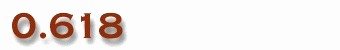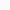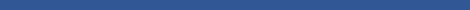Epsilon is a library of functions implemented in Maple and Java for polynomial elimination and decomposition with (geometric) applications. It has 8 modules and contains more than 70 functions, which allow one to

• triangularize systems of multivariate (differential) polynomials,
• decompose polynomial systems into triangular systems of various kinds (regular, normal, simple, irreducible, or with projection property),
• decompose algebraic varieties into irreducible or unmixed subvarieties,
• decompose polynomial ideals into primary components,
• factorize polynomials over algebraic extension fields,
• solve systems of polynomial equations and inequations, and
• handle and prove geometric theorems automatically.
The entire library with documentation, examples, and Maple worksheets are available at